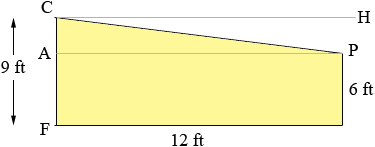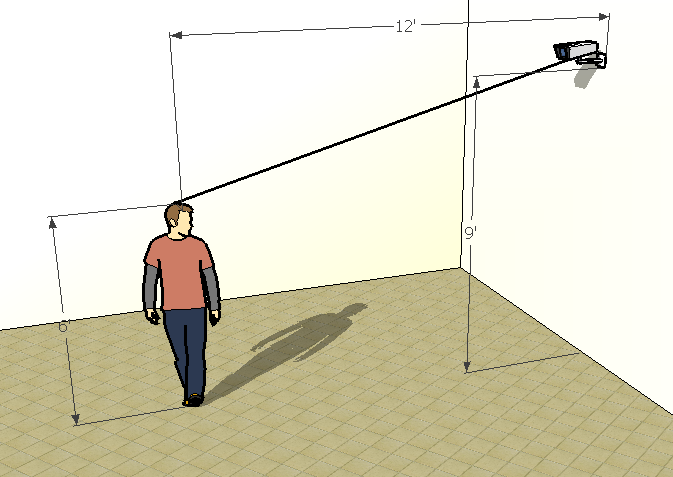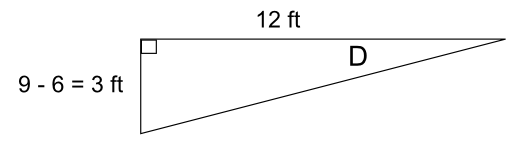SEARCH HOMEMath Central Quandaries & QueriesQuestion from Rita, a student: A security camera in a neighborhood carnival is mounted on a wall 9 feet above the floor inside a video gallery. What angle of depression should be used if the camera is to be directed to a spot 6 feet above the floor and 12 feet from the wall? Thank you very much.We have two responses for you

Rita,The camera is at C, The floor at F, the point at which the camera is directed is P and CH is a horizontal line. The angle of depression is the angle HCP.

Harley

Hi Rita. Take a look at this sketch:Here it is boiled down to the geometries involved (I hope you can see how the geometric diagram comes from the sketch):Can you see what trig function lets you calculate the angle of depression (D in my diagram) in this triangle?

Cheers,
Stephen La RocqueMath Central is supported by the University of Regina and The Pacific Institute for the Mathematical Sciences.